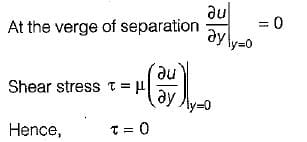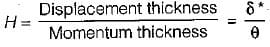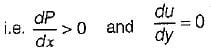# Test: Boundary Layer Theory - 2

## 10 Questions MCQ Test GATE Civil Engineering (CE) 2023 Mock Test Series | Test: Boundary Layer Theory - 2

Description
Attempt Test: Boundary Layer Theory - 2 | 10 questions in 30 minutes | Mock test for GATE preparation | Free important questions MCQ to study GATE Civil Engineering (CE) 2023 Mock Test Series for GATE Exam | Download free PDF with solutions
QUESTION: 1

Solution:
QUESTION: 2

### It is recommended that the diffuser angle should be kept less than 18° because

Solution:

If diffuser angle is more, eddies formation may takes place and also flow separation may occurs.

QUESTION: 3

### Boundary layer separation is caused by

Solution:

QUESTION: 4

Laminar sub-layer acts as

Solution:

In turbulent flow analysis along the boundary layer, a thin layer of fluid in the immediate neighbourhood of the boundary where viscous shear stress is predominant while the shear stress due to turbulence is negligible. This portion is known as laminar sublayer.
In turbulent flow heat transfer is more as compared to laminar flow and also is this laminar sublayer heat transfer is very less while, it is present is turbulent flow hence it acts as insulating medium in turbulent flow.

QUESTION: 5

At the point of boundary layer separation

Solution:QUESTION: 6

If u = free stream velocity
u = local velocity.
δ = boundary layer thickness
Then the momentum thickness θ is given by

Solution:
QUESTION: 7

The energy thickness δe is given by

Solution:
QUESTION: 8

The displacement thickness δ* is

Solution:
QUESTION: 9

Given that
δ = boundary layer thickness
δ* = displacement thicknes
δe = energy thickness
θ = momentum thickness
The shape factor H is given by

Solution:

Shape factor is given byQUESTION: 10

Boundary layer flow separates from the surface if

Solution:Use Code STAYHOME200 and get INR 200 additional OFF Use Coupon Code